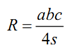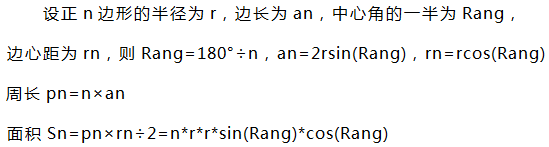#Codeforces Beta Round #1 A,B,C

0
0
01. 云栖社区>
2. 博客>
3. 正文

## Codeforces Beta Round #1 A,B,C

angel_kitty 2017-03-10 23:24:00 浏览359

A. Theatre Square
time limit per test:1 second
memory limit per test:256 megabytes
input:standard input
output:standard output

Theatre Square in the capital city of Berland has a rectangular shape with the size n × m meters. On the occasion of the city's anniversary, a decision was taken to pave the Square with square granite flagstones. Each flagstone is of the size a × a.

What is the least number of flagstones needed to pave the Square? It's allowed to cover the surface larger than the Theatre Square, but the Square has to be covered. It's not allowed to break the flagstones. The sides of flagstones should be parallel to the sides of the Square.

Input

The input contains three positive integer numbers in the first line: n,  m and a (1 ≤  n, m, a ≤ 109).

Output

Write the needed number of flagstones.

Examples
Input
`6 6 4`
Output
`4`

``` 1 #include <bits/stdc++.h>
2 using namespace std;
3 int main()
4 {
5     __int64 n,m,a;
6     while(scanf("%I64d%I64d%I64d",&n,&m,&a)!=EOF)
7     {
8         __int64 t=n/a;
9         __int64 s=m/a;
10         if(n%a!=0)
11             t+=1;
12         if(m%a!=0)
13             s+=1;
14         __int64 k=t*s;
15         printf("%I64d\n",k);
16     }
17     return 0;
18 }```
time limit per test：10 seconds
memory limit per test：64 megabytes
input：standard input
output：standard output

In the popular spreadsheets systems (for example, in Excel) the following numeration of columns is used. The first column has number A, the second — number B, etc. till column 26 that is marked by Z. Then there are two-letter numbers: column 27 has number AA, 28 — AB, column 52 is marked by AZ. After ZZ there follow three-letter numbers, etc.

The rows are marked by integer numbers starting with 1. The cell name is the concatenation of the column and the row numbers. For example, BC23 is the name for the cell that is in column 55, row 23.

Sometimes another numeration system is used: RXCY, where X and Y are integer numbers, showing the column and the row numbers respectfully. For instance, R23C55 is the cell from the previous example.

Your task is to write a program that reads the given sequence of cell coordinates and produce each item written according to the rules of another numeration system.

Input

The first line of the input contains integer number n (1 ≤ n ≤ 105), the number of coordinates in the test. Then there follow n lines, each of them contains coordinates. All the coordinates are correct, there are no cells with the column and/or the row numbers larger than 106 .

Output

Write n lines, each line should contain a cell coordinates in the other numeration system.

Examples
Input
`2 R23C55 BC23`
Output
`BC23R23C55`

①R23C55

②BC23

1-26列：A, B, C...Z

27-?列：AA, AB, AC...AZ, BA, BB, BC...ZZ

?-?：AAA...ZZZ...

``` 1 #include <bits/stdc++.h>
2 using namespace std;
3 const int maxn=1000005;
4 typedef long long ll;
5 char str[maxn];
6 int flag;
7 void change(int n)//26进制转换
8 {
9     if(n>26)
10         change((n-1)/26);
11     printf("%c",(n-1)%26+'A');
12 }
13 void solve1()
14 {
15     int row=0;
16     int col=0;
17     for(int i=1;i<flag;i++)
18         if(isdigit(str[i]))
19         row=row*10+str[i]-'0';//计算行
20     for(int i=flag+1;str[i];i++)
21         col=col*10+str[i]-'0';//计算列
22     change(col);
23     printf("%d\n",row);
24 }
25 void solve2()
26 {
27     int row=0;
28     int col=0;
29     for(int i=0;str[i];i++)
30     {
31         if(isupper(str[i]))
32             col=col*26+str[i]-'A'+1;//计算列
33         else row=row*10+str[i]-'0';//计算行
34     }
35     printf("R%dC%d\n",row,col);
36 }
37 int main()
38 {
39     int T;
40     while(scanf("%d",&T)!=EOF)
41     {
42         while(T--)
43         {
44             scanf("%s",str);
45             flag=0;
46             if(str=='R'&&isdigit(str))
47             {
48                 for(int i=2;str[i];i++)
49                 {
50                     if(str[i]=='C')
51                     {
52                         flag=i;
53                         break;
54                     }
55                 }
56             }
57             if(flag)
58                 solve1();//判断‘R23C55’这一种情况
59             else
60                 solve2();//判断‘BC23’这一种情况
61         }
62     }
63     return 0;
64 }```
C. Ancient Berland Circus
time limit per test：2 seconds
memory limit per test：64 megabytes
input：standard input
output：standard output

Nowadays all circuses in Berland have a round arena with diameter 13 meters, but in the past things were different.

In Ancient Berland arenas in circuses were shaped as a regular (equiangular) polygon, the size and the number of angles could vary from one circus to another. In each corner of the arena there was a special pillar, and the rope strung between the pillars marked the arena edges.

Recently the scientists from Berland have discovered the remains of the ancient circus arena. They found only three pillars, the others were destroyed by the time.

You are given the coordinates of these three pillars. Find out what is the smallest area that the arena could have.

Input

The input file consists of three lines, each of them contains a pair of numbers –– coordinates of the pillar. Any coordinate doesn't exceed 1000 by absolute value, and is given with at most six digits after decimal point.

Output

Output the smallest possible area of the ancient arena. This number should be accurate to at least 6 digits after the decimal point. It's guaranteed that the number of angles in the optimal polygon is not larger than 100.

Examples
Input
`0.000000 0.000000 1.000000 1.000000 0.000000 1.000000`
Output
`1.00000000`
题目链接：http://codeforces.com/problemset/problem/1/C下面给出AC代码：
``` 1 #include <bits/stdc++.h>
2 using namespace std;
3 const double PI=3.1415926535;
4 const double ERR=0.01;
5 bool feq(double a,double b)
6 {
7     return fabs(a-b)<ERR;
8 }
9 double fgcd(double a,double b)
10 {
11     if (feq(a,0))
12         return b;
13     if (feq(b,0))
14         return a;
15     return fgcd(b,fmod(a,b));
16 }
17 double dist(double x0,double x1,double y0,double y1)
18 {
19     return sqrt((x1-x0)*(x1-x0)+(y1-y0)*(y1-y0));
20 }
21 int main()
22 {
23     double x;
24     double y;
25     for (int i=0;i<3;i++)
26         scanf("%lf%lf",&x[i],&y[i]);
27     double l;
28     for (int i=0;i<3;i++) l[i]=dist(x[i],x[(i+1)%3],y[i],y[(i+1)%3]);
29     double p=(l+l+l)/2;
30     double s=sqrt(p*(p-l)*(p-l)*(p-l));
31     double r=l*l*l/(s*4);
32     double ang;
33     for (int i=0;i<3;i++) ang[i] = acos(1-l[i]*l[i]/(2*r*r));
34     ang =2*PI-ang-ang;
35     double unita =0;
36     for (int i=0;i<3;i++)
37         unita=fgcd(unita,ang[i]);
38     printf("%.6lf\n",r*r*sin(unita)*PI/unita);
39     return 0;
40 }```

angel_kitty
+ 关注